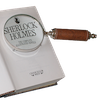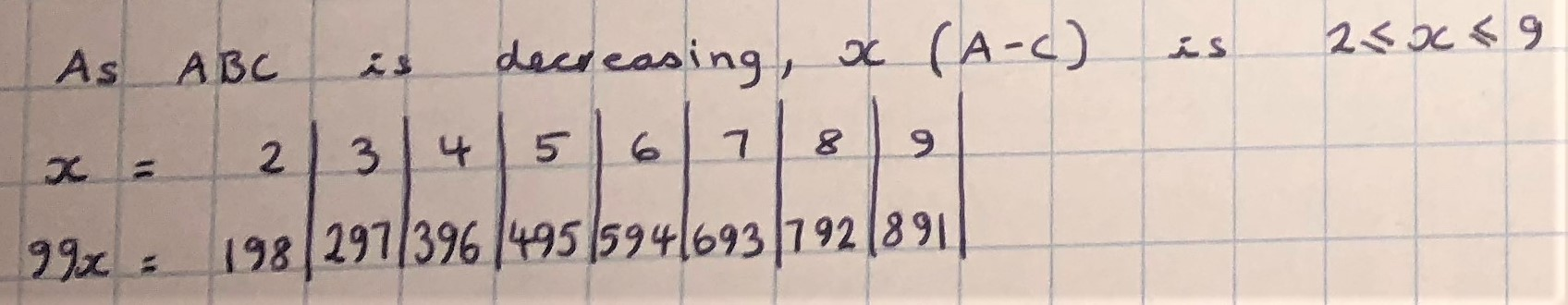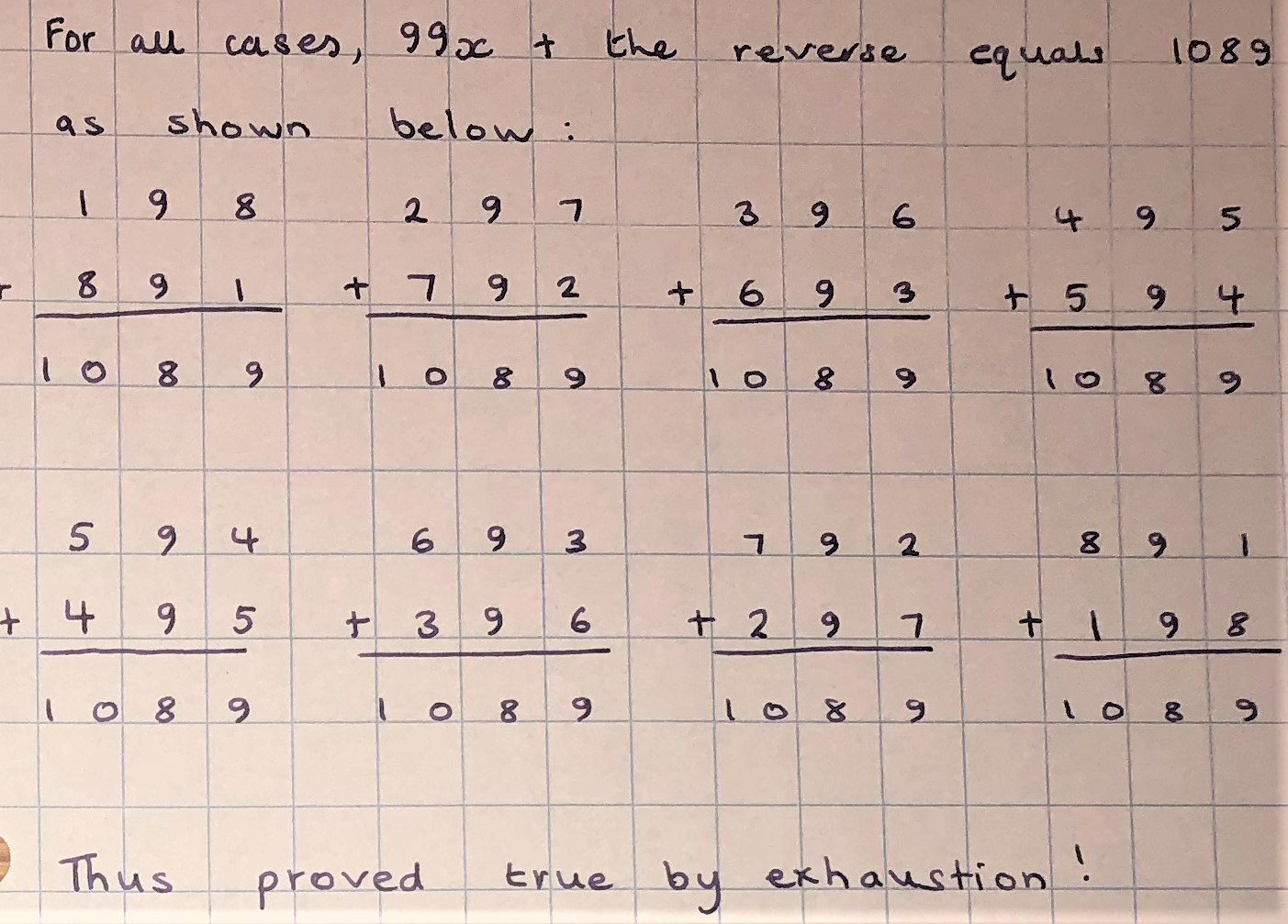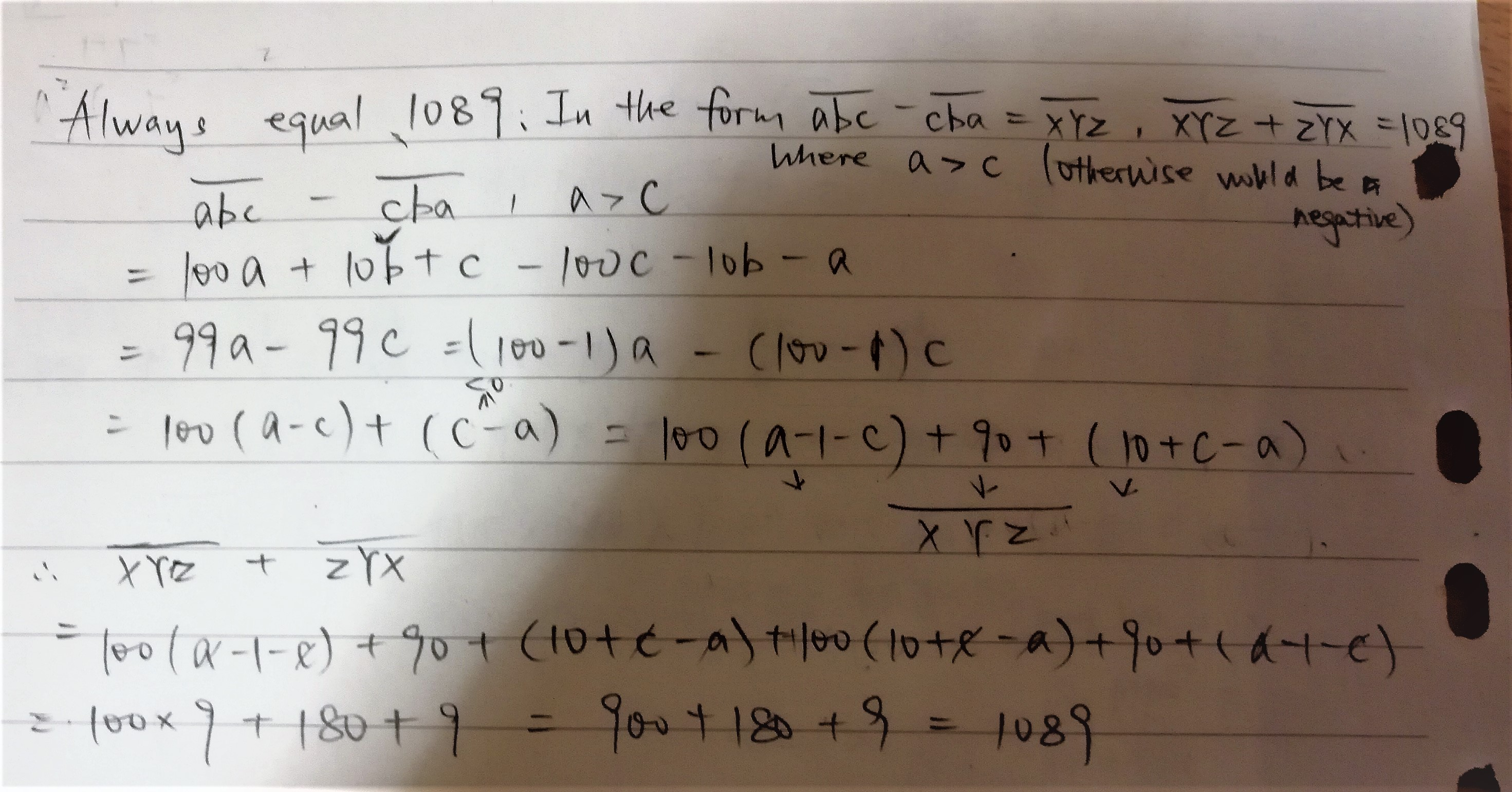#### You may also like### Double Digit### Number Detective

Follow the clues to find the mystery number.### Six Is the Sum

What do the digits in the number fifteen add up to? How many other numbers have digits with the same total but no zeros?

# Subtraction Surprise

##### Age 7 to 14Challenge Level

Here are some examples that were sent in:
Name Step 1 Step 2 Comments
Abdullah 971 - 179 = 792
900 - 009 = 891
792 + 297 = 1098
891 + 198 = 1089
All the subtraction results are
a multiple of 99.
The mutliples of 99 are
reverse of each other.
Manahal 967 - 769 = 198 198 + 891 = 1089 Your answer should be 1089
Laxmi 594 - 495 = 99 099 + 990 = 1089 The total will always come to
1089
Hayley 863 - 368 = 495 495 + 594 = 1089 4 digit numbers have an
outcome of 10890
2 digit numbers have an
outcome of 99

Amit from Lester B. Pearson School Of The Arts noticed that it doesn't work for 111, 222, 333, 444, 555, 666, 777, 888 or 999. Aaron from St Stephens School built on this:
If you have a palindromic, three digit number, and you reverse it and subtract the reversed number from the original number, the [result] will be zero. If you do the second step of reversing and adding, it will be zero again. It will not be 1089.

My conjecture is that the answer to the reverse and subtract procedure is 1089 only if the number is not palindromic. If it is, then the answer to the procedure will be zero.

Lucas from Powers Hall Academy in the UK, Jasmine and Ella from Cliffedale in the UK and Jack from Chandlings explained why the result is usually 1089 by looking at the sums. This is a mixture of their explanations:
Lucas: If you look, the 1st and 3rd digit cannot be the same, so the smaller number will have a larger 1s digit (as the larger number must have the larger 100s digit). You have to borrow. (998 reverse is 899. As you can see 998 has a smaller 1s digit than 899).

Jasmine and Ella: The tens digits (of the subtraction) are always the same and so [when] you borrow from the tens column, [you make] the top tens digit one less.

Then you have to borrow from the hundreds column, making [the top tens digit] nine more than the bottom tens digit, so when you take the bottom tens digit (of the subtraction) off of the top tens digit (of the subtraction) you will get nine.

Jack: When you swap them around to add the answer together, the hundreds and ones will always be number bonds to nine and when you add nine  and nine it equals eighteen so you carry the one and when you add nine and one it equals ten which is why the answer is 1089

The only part that is still unexplained is why the first and third digits after the subtraction will be number bonds to 9.

Dylan from Gahanna Middle School South used the same technique, but with letters rather than numbers:

First, we have a three digit number with the reversal of it subtracted from it.
A    B    C
$-$ C    B    A
Since C is less than A we have to take one from B

A   B-1  C+10
$-$ C    B     A

Since B-1 is less than B we will have to take 1 from A and add ten to B-1.

A-1    B+9    C+10
$-$  C       B        A

B+9-B=9 so the middle digit is always a nine.

In the experiment, you realize that the first and last digit of the first answer add up to nine
Example: 543-345=198 --> 1 and 8 add up to nine

So that means that (A-1-C)+(C+10-A)=9
That simplified is this A-1-C+C+10-A=9
That simplified is  A-A+C-C+9=9
That simplified is 9=9
That means any value of A or C will, in this equation
A  B  C
- C  B  A
when added up on both sides will equal

D 9 E          (D + E = 9)

D  9  E
+ E  9  D

Since E+D=9 the left value is 9

D  9  E
+ E  9  D
9
9+9=18 so the middle value is 8 with the carried 1

D+1 9  E
+  E   9  D
8  9

Since D + E = 9
Then D + 1 + E = 10
D+1 9  E
+ E    9  D
1 0   8  9

No matter what A B or C is the value after the changes will always be 1089

Aanya from Arnhem Wharf Primary School in the UK, Kian from King Edward VII School in the UK, Freya from St Simon Stock in the UK and Matthew from George Watson's College used more formal algebra which led them to the 99 times table. This is the beginning of Matthew's work:
The sum is ABC $-$ CBA
ABC has an A in the hundreds column so is effectively 100A
It also has a B in the tens column so is basically 10B
And the C in the units column is just one C

CBA is 100C, 10B and A
100A$-$A is 99A, 10B$-$10B is 0 and C$-$100C is $-$99C
So the answer is 99A$-$99C
99A$-$99C is a multiple of 99 under 11 (in fact under 9, since A and C are both 9 or less)

Freya and Aanya checked all of the small multiples of 99 to show that the final answer will always be 1089. This is Freya's work:(It was also necessary to check the case A$-$C = 1, 1$\times$99 = 99 or 099, 099 + 990 = 1089).

Matthew and Kian also looked at all of the small multiples of 99. Kian noticed the pattern that was described above by Lucas, Jasmine and Ella and Jack:

Notice that in all of these, the first and last digits sum to 9, and the middle digit is always 9.
Aanya explained why the first and last digits sum to 9:
We also know that 99x$-$99z is divisible by 9 because it could be written as 9(11x$-$11z). So we know that the last digit and the first digit add up to 9, because for all numbers divisible by nine the sum of the digits is nine.

Matthew said:
All multiples of 99 under 11 are still multiples of 99 under 11 if you write them backwards
And they add up to 11x99 which is 1089
(that is, $99\times a$ is the reverse of $99\times(11-a)$ for $a$ a whole number up to $11$. And $99\times a + 99\times(11-a) = 99\times11=1089) Reyna from Downside School used algebra in this way, but a method similar to Dylan's. Notice that Renya points out that$c-a<0$, and this is how the numbers are regrouped for the column subtraction:Johnny from Malvern College Chengdu in China used similar algebra, but instead of using a different letter for each digit, used only two different letters at a time. This meant Johnny needed to test out different cases, and each case corresponds to a multiple of 99. Click here to see Johnny's work. The year 9 top set at British International School Phuket continued the investigation for 4 and 5 digit numbers: 4 digit numbers Let the 4 digit number$abcd$(with$a > d$) be represented as$1000a + 100b + 10c + d.$Therefore the 4 digit number$dcba$is represented as$1000d + 100c + 10b + a.$$$\begin{split}1000a + 100b + 10c + d - (1000d + 100c + 10b + a) &= 999a + 90b - 90c -999d\\ &= 999(a-d) + 90(b-c) \end{split}$$ Let's look for a pattern with say$a = 4, b = 3, c = 2, d =1.$This gives$3087.$So we can get$7803$by doing: $$999(10 - (a-d)) + 90(10 -(b-c))$$ Let's check that this works with another number:$a = 6, b = 5, c = 4, d = 1.$Gives$5085.Which also works for our formula. So we hypothesize that this is the correct formula. Therefore: \begin{align}999a + &90b - 90c -999d + ( 999(10 - (a-d)) + 90(10 -(b-c)) )\\ &=999a + 90b - 90c -999d + 9990-999a+999d+900-90b+90c \\&= 9990+ 900 = 10890\end{align} 5 digit numbers Using the same method we find that $$9999a + 990b - 990d -999e + ( 9999(10 - (a-e)) + 990(10 -(b-d)) ) = 109890$$ Therefore for 3 digits we have:99(11) = 99(10) + 9(10) + 9 = 1089$For 4 digits we have:$999(10) + 90(10)  = 10890$For 5 digits we have:$9999(10) + 999(10) - 9(10) = 109890\$